# Survival tests as differences-of-means

## A simple randomized controlled trial

Consider a simple randomized controlled trial in which $$N$$ patients are randomized between treatment $$1$$ and treatment $$0$$ (let $$Z_i$$ denote treatment assignment for patient $$i$$). Each patient contributes a response $$Y_i$$, and we are primarily interested in the difference in means on the two treatments,

$S:= \frac{\sum_{i=1}^N Y_i \mathbb{I}(Z_i = 1)}{\sum_{i=1}^N \mathbb{I}(Z_i = 1)} - \frac{\sum_{i=1}^N Y_i \mathbb{I}(Z_i = 0)}{\sum_{i=1}^N \mathbb{I}(Z_i = 0)}.$

If this statistic is large then we would consider that evidence in favour of treatment $$A$$.

## Time-to-event outcome

If we have a time-to-event outcomes $$(T_i, \delta_i)$$, where $$T_i = \min(\tilde{T}_i, C_i)$$ is the follow-up time (the minimum of the time-to-event-of-interest and time-to-censoring) and $$\delta_i = \mathbb{I}\{\tilde{T}_i < C_i\}$$, then since the outcome is two dimensional we cannot simply compare the treatments via a difference in means.

## …Unless

Unless we first make a transformation of the two-dimensional outcome space to a one-dimensional “score”, and then compare the mean “score” on the two arms. It turns out that a large number of test statistics that are commonly used in time-to-event settings can be expressed in this way:

• log-rank statistics
• weighted log-rank statistic
• difference in restricted mean survival times
• difference in milestone survival

## What do the scores look like?

Let’s take the example of the log-rank statistic applied to the data from the POPLAR study (Gandara et al.).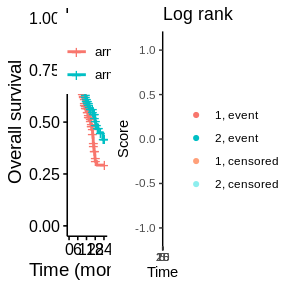In the graph on the right-hand side, each dot corresponds to a patient in the trial. On the x-axis is the patients’ follow-up time and on the y-axis is the score assigned to each observation. The dots form two approximately parallel lines. The top line corresponds to observed events; the bottom line corresponds to censored observations. An observed event close to time zero receives a score close to 1; a censored observation at around month 24 gets a score of -1; intermediate outcomes receive an intermediate score (the scores have been shifted and scaled to range between 1 and -1). The mean score on the two treatment arms are indicated with horizontal lines. The difference in mean score is the log-rank statistic (or, more precisely, a re-scaled version of it).

## Why is this interesting?

I think this perspective becomes helpful when we start to compare alternative test statistics. One popular approach in the context of non-proportional hazards is to base inference on the difference in restricted mean survival times (RMST) on the two arms (based on the Kaplan-Meier estimates). If we express the corresponding test statistic as a difference in scores (following Andersen et el.), we see that every observation after the restriction time (in this case 18 months) get the same score. So an observed event at month 18 receives the same score as a censored observation at 24 months, for example.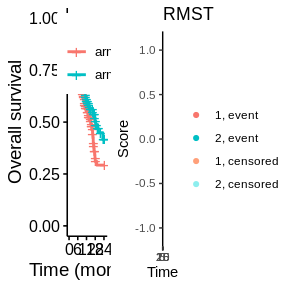Next we might try a statistic based on the difference in milestone survival probabilities at 12 months (via the Kaplan-Meier estimates). If there were no censoring prior to 12 months, this would be like giving everyone with an event prior to 12 months a score of 1 and everyone still alive at 12 months a score of -1. Owing to censoring, however, events closer to 12 months are up-weighted.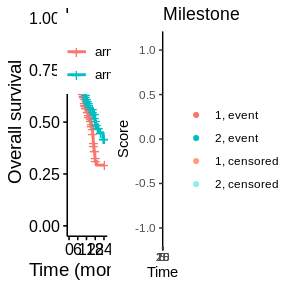## Weighted log-rank tests

Weighted log-rank tests can also be compared in this framework. For example, a modestly-weighted log-rank test ($$t^*= 9$$).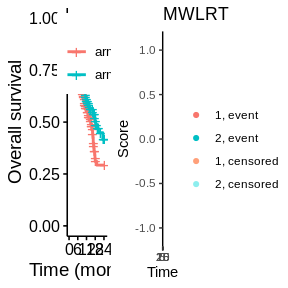This might be thought of as intermediate between a log-rank test and a milestone test. It’s similar to a milestone analysis in the sense that it is a contrast of the later parts of the survival curves, with early events all getting a score of 1. However, unlike the milestone analysis, the contrast does not focus on just one timepoint. Rather, it is an average contrast over late follow-up times with better outomes receiving gradually better scores, and in this sense is more like the log-rank test.

## Early versus late contrasts

By looking carefully at these graphs we can get a sense about whether a particular test is putting more emphasis on early parts of follow-up or late parts of follow-up. Roughly speaking, if, at a particular Time, there is a large difference betwen the score given to an observed event and the score given to a censored observation, then this indicates that heavy emphasis is being given to that timepoint. Here, for example, we see that for RMST the scores give high emphasis to early follow-up times, and vice-versa for the milestone and modestly-weighted tests. The log-rank test has more gradually changing scores across the whole follow-up period.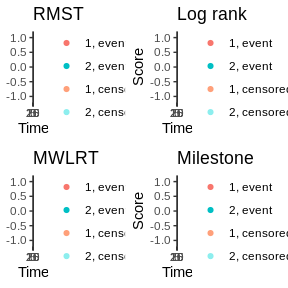# Appendix

## Where do these numbers come from?

In all cases, we can think in the permutation test framework, considering the scores as fixed and permuting the treatment labels.

### (Weighted) log-rank test

Following Leton & Zuluaga (2000), letting $$l_{1,j}$$ and $$l_{0,j}$$ denote the number of patients censored on the test treatment and control treatment, respectively, during $$\left[\left.t_j, t_{j+1}\right)\right.$$, we can express the (weighted) log-rank statistic as

\begin{align*} U_W &:=\sum_{j = 1}^{k} w_j\left( O_{1,j} - O_j\frac{n_{1,j}}{n_j} \right)\\ &=\sum_{j = 1}^{k} w_jO_{1,j} - \sum_{j = 1}^{k}w_j\frac{O_{j}}{n_j} \times n_{1,j}\\ &=\sum_{j = 1}^{k} w_jO_{1,j} - \sum_{j = 1}^{k}w_j\frac{O_{j}}{n_j} \times \sum_{i = j}^{k}(O_{1,i} + l_{1,i})\\ &=\sum_{j = 1}^{k} w_jO_{1,j} - \sum_{j = 1}^{k}(O_{1,j} + l_{1,j}) \times \sum_{i = 1}^{j}w_i\frac{O_{i}}{n_i}\\ &=\sum_{j = 1}^{k} O_{1,j}\left( w_j - \sum_{i = 1}^{j}w_i\frac{O_{i}}{n_i} \right) + \sum_{j = 1}^{k}l_{1,j} \left( - \sum_{i = 1}^{j}w_i\frac{O_{i}}{n_i}\right). \end{align*}

This means that an observed event at time $$t_j$$ is given a score of $$a_i=w_j - \sum_{i = 1}^{j}w_i\frac{O_{i}}{n_i}$$, and an observation censored during $$\left[\left.t_j, t_{j+1}\right)\right.$$ is given a score of $$a_i=- \sum_{i = 1}^{j}w_i\frac{O_{i}}{n_i}$$.

Note that instead of using $$U_W$$ one could also use $\begin{equation*} \tilde{U}_W = \frac{\sum_{i= 1}^n \mathbb{I}\left\lbrace z_{i} = 1 \right\rbrace a_{i}}{\sum_{i= 1}^n \mathbb{I}\left\lbrace z_{i} = 1 \right\rbrace} - \frac{\sum_{i= 1}^n \mathbb{I}\left\lbrace z_{i} = 0 \right\rbrace a_{i}}{\sum_{i= 1}^n \mathbb{I}\left\lbrace z_{(i)} = 0 \right\rbrace}, \end{equation*}$ as the test statistic in a permutation test, as $$U$$ and $$\tilde{U}$$ are equivalent up to a (positive) scale and shift transformation, i.e., $\begin{equation*} \tilde{U}_W = \frac{n}{\sum_{i= 1}^n \mathbb{I}\left\lbrace z_{i} = 1 \right\rbrace\sum_{i= 1}^n \mathbb{I}\left\lbrace z_{i} = 0 \right\rbrace} \times U_W - \frac{\sum_{i= 1}^n a_{i} \sum_{i= 1}^n \mathbb{I}\left\lbrace z_{i} = 1 \right\rbrace}{\sum_{i= 1}^n \mathbb{I}\left\lbrace z_{i} = 1 \right\rbrace\sum_{i= 1}^n \mathbb{I}\left\lbrace z_{i} = 0 \right\rbrace}. \end{equation*}$

Furthermore, re-scaling the scores to

$b_i = \frac{2a_i - \max{a} - \min{a}}{\max{a} - \min{a}}$ so that $$b \in (-1,1)$$ would also leave the p-value of the permutation test unchanged.

### RMST / Milestone

We use the concept of pseudo-values following Andersen et al. (2017).

For the RMST, without any adjustment for covariates, the $$i$$-th pseudo-value (at time $$\tau$$) is defined as $\widehat{\theta}_{i}^{\tiny \mbox{RMST}} = n \int_0^\tau \widehat{S}(t) dt - (n-1) \int_0^\tau \widehat{S}^{(-i)}(t) dt,$ where $$\widehat{S}^{(-i)}(t)$$ is the Kaplan-Meier estimator excluding observation (or subject) $$i$$. For the Milestone survival analysis rate, also without any adjustment for covariates, the $$i$$-th pseudo-value is defined as $\widehat{\theta}_{i}^{\tiny \mbox{MLST}} = n \widehat{S}(t) - (n-1) \widehat{S}^{(-i)}(t).$ Having found pseudo-values for each patient, they can be used just like any other (continuous) outcomes. For example, they could be fed into a linear model with treatment term only. In this case, the resultant test statistic for testing the null hypothesis of zero difference between treatments would be a difference in mean pseudo-values, i.e., the pseudo-values are performing the same role as the “scores” in the weighted log-rank tests. Again these scores could be standardized to lie between -1 and 1 without affecting the p-value.